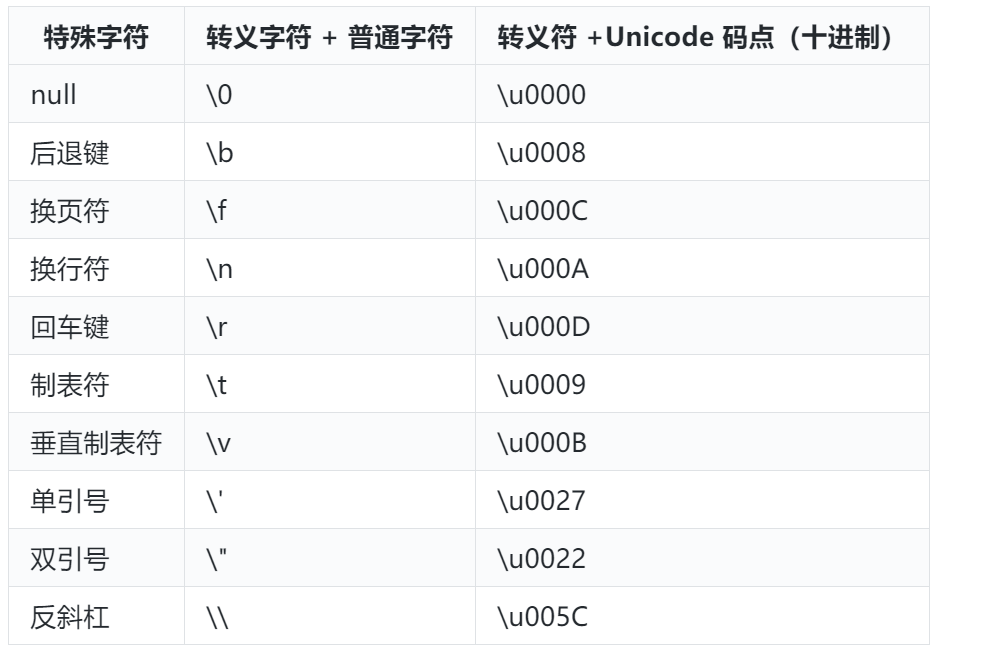# JavaScript 的类型系统

0收藏

Null 和 Undefined 类型

``````Number(null) // 0
5 + null // 5``````

``````Number(undefined) // NaN
5 + undefined // NaN``````

Number 类型

``1 === 1.0 // true``

``````// 十进制：无前缀，或者有前缀0但是后面有8或9
var num1 = 21;
var num2 = 0789; // 这是十进制，因为这里有8和9

// 八进制：
// 使用前缀0表示（ES5的严格模式不再允许使用该写法）。
var num3 = 0123;
// 使用前缀0o或0O表示（ES6引入的新写法）。
var num4 = 0o123;

// 十六进制：使用前缀0x或0X表示。
var num5 = 0xff;

// 科学计数法
var num6 = 25e2; // 2500
var num7 = 25e-2; // 0.25

// 二进制：使用前缀0b或0B表示（ES6引入的新写法）。
var num8 = 0b111110111; // 503
``````

NaN

NaN 表示“非数字”（not a number），主要用在将字符串解析成数值出错等场合。

``````5 - 'x' // NaN
0 / 0 // NaN``````

Infinity

Infinity 表示“无穷”，用来表示两种场景。一种是一个正的数值太大，或一个负的数值太小，无法表示；另一种是非 0 数值除以 0，得到 Infinity。
Infinity 有正负之分，Infinity 表示正的无穷，-Infinity 表示负的无穷。

Bigint 类型

JavaScript 所有数字都保存成 64 位浮点数，这给数值的表示带来了两大限制。一是数值的精度只能到 53 个二进制位（相当于 16 个十进制位），大于这个范围的整数，JavaScript 是无法精确表示的，这使得 JavaScript 不适合进行科学和金融方面的精确计算。二是大于或等于 2 的 1024 次方的数值，JavaScript 无法表示，会返回 Infinity。

``````// 超过 53 个二进制位的数值，无法保持精度
Math.pow(2, 53) === Math.pow(2, 53) + 1 // true

// 超过 2 的 1024 次方的数值，无法表示
Math.pow(2, 1024) // Infinity
``````

ES2020 引入了一种新的数据类型 BigInt（大整数），来解决这个问题，这是 ECMAScript 的第八种数据类型。BigInt 只用来表示整数，没有位数的限制，任何位数的整数都可以精确表示。

``````const a = 2172141653n;
const b = 15346349309n;

// BigInt 可以保持精度
a * b // 33334444555566667777n``````

String 类型

``````// 字符串需放在单引号或者双引号之中。
var str1 = 'abc';
var str2 = "abc";

// 单引号字符串内部，可以使用双引号。双引号字符串内部可以使用单引号。
var str1 = 'key = "value"'
var str2 = "It's a long journey"

// 在单（双）引号字符串内部使用单（双）引号，需用反斜杠来进行转义。
var str1 = 'Did she say \'Hello\'?'
var str2 = "Did she say \"Hello\"?"

// 当字符串太长时，可以使用反斜杠进行折行，效果与写在同一个行完全一样。
// 需注意的是：
//    1. 从第二行开始，不要添加多余的空格，否则这些空格也会成为字符串的一部分。
//    2. 反斜杠后边必须是换行符，不能有其他字符（比如空格）。
var longString = 'Long \
long \
long \
string';
``````

``````// 使用拼接符
\$('#result').append(
'There are <b>' + basket.count + '</b> ' +
'</em> are on sale!'
);
// 使用模板字符串
\$('#result').append(`
are on sale!
`);

``````

• 转义符（反斜杠）+ 普通字符
• 转义符 + Unicode 码点length 属性返回字符串的长度，该属性也是无法改变的。

``````var s = 'hello';
s.length // 5

s.length = 3;
s.length // 5``````

JavaScript 使用 Unicode 字符集。每个字符在 JavaScript 内部都是以 16 位（即 2 个字节）的 UTF-16 格式储存。

Symbol 类型

ES5 的对象属性名都是字符串，这容易造成属性名的冲突。比如，你使用了一个他人提供的对象，但又想为这个对象添加新的方法，新方法的名字就有可能与现有方法产生冲突。为了从根本上防止属性名的冲突，ES6 引入了 Symbol 类型。

Symbol 类型是一种新的原始数据类型，它表示独一无二的值。Symbol 值可以用作对象的属性名

Symbol 值通过 Symbol 函数生成。该值不是对象，因此 Symbol 函数前不能使用 new 命令，否则会报错。

Symbol 函数可以接受一个字符串作为参数，表示对 Symbol 实例的描述，主要是为了在控制台显示，或者转为字符串时，比较容易区分。该参数可以是原始类型，也可以是对象，并且不会对 Symbol 值产生影响。

``````let s1 = Symbol('foo');
let s2 = Symbol('bar');

s1 // Symbol(foo)
s2 // Symbol(bar)

s1.toString() // "Symbol(foo)"
s2.toString() // "Symbol(bar)"

let s3 = Symbol('foo');
s1 === s3 // false

``````

Symbol 值可以显式转为字符串或者布尔值：

``````let sym = Symbol('My symbol');

String(sym) // 'Symbol(My symbol)'
sym.toString() // 'Symbol(My symbol)'

let sym = Symbol();
Boolean(sym) // true
!sym  // false

``````

Symbol 值作为对象属性名时，不能用点运算符，只能使用方括号。

``````let mySymbol = Symbol();

// 第一种写法
let a = {};
a[mySymbol] = 'Hello!';

// 第二种写法
let a = {
[mySymbol]: 'Hello!'
};

// 第三种写法
let a = {};
Object.defineProperty(a, mySymbol, { value: 'Hello!' });

// 以上写法都得到同样结果
a[mySymbol] // "Hello!"
``````

Object 类型

``````// 这是一个空的对象
var obj = {};

// 创建一个Student对象
var student = {
name: 'Tom',
age: 12
}``````

JavaScript 语言使用构造函数（constructor）作为对象的模板，通过 new 命令执行构造函数来生成实例对象。比如：Object 就是一个构造函数。

``````// 创建一个空的对象
var obj = new Object();

// 定义一个Student构造函数
var Student = function(){
this.name = 'Tom',
this.age = 12
};
// 创建一个Student对象实例
var s = new Student();

``````

Class（类）

ES6 引入了 Class（类）这个概念，作为对象的模板，通过 class 关键字，可以定义类。

``````// 定义一个Student类
class Student {
// 构造方法，生成对象实例时被调用
constructor(name, age) {
this.name = name;
this.age = age;
}
// 定义“类”的方法时，前面不需要添加function关键字
toString() {
return '(name: ' + this.name + ', age: ' + this.age + ')';
}
}
// 创建一个Student对象实例
var s = new Student('Tom', 12);
``````

ECMAScript 规范明确定义了各种对象的类别，包括：

• 常规对象（ordinary object）拥有 JavaScript 对象所有的默认行为。
• 特异对象（exotic object）的某些内部行为和默认的有所差异。
• 标准对象（standard object）是 ECMAScript 6 中定义的对象，例如 Array, Date 等，它们既可能是常规也可能是特异对象。
• 内置对象（built-in object）指 JavaScript 执行环境开始运行时已存在的对象。标准对象均为内置对象。

``````typeof 123; // 'number'
typeof NaN; // 'number'
typeof 'str'; // 'string'
typeof true; // 'boolean'
typeof undefined; // 'undefined'
typeof Math.abs; // 'function'，函数也是对象
typeof null; // 'object'
typeof []; // 'object'
typeof {}; // 'object'``````

null 的类型是 object，这是由于历史原因造成的。1995 年的 JavaScript 语言第一版，只设计了五种数据类型（对象、整数、浮点数、字符串和布尔值），没考虑 null，只把它当作 object 的一种特殊值。后来 null 独立出来，作为一种单独的数据类型，为了兼容以前的代码，typeof null 返回 object 就没法改变了。